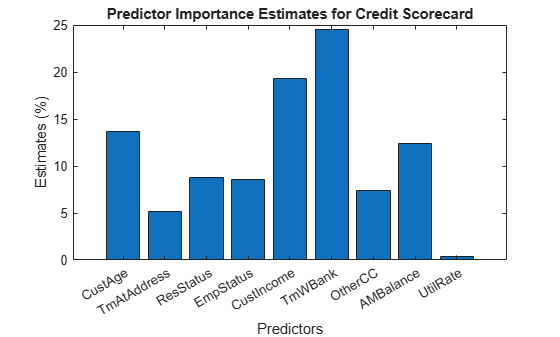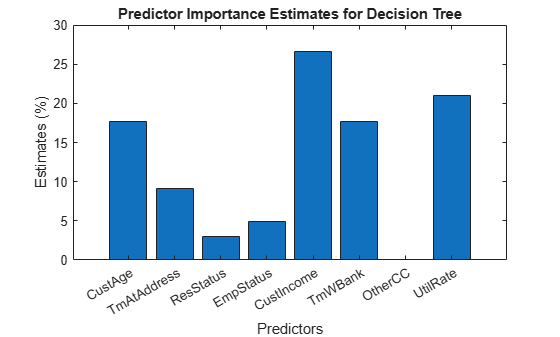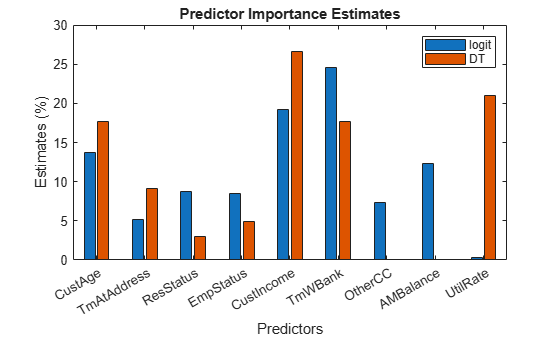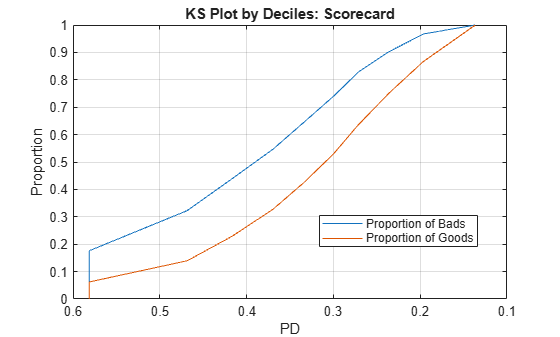Comparison of Credit Scoring Using Logistic Regression and Decision Trees

This example shows the workflow for creating and comparing two credit scoring models: a credit scoring model based on logistic regression and a credit scoring model based on decision trees.

Credit rating agencies and banks use challenger models to test the credibility and goodness of a credit scoring model. In this example, the base model is a logistic regression model and the challenger model is a decision tree model.

Logistic regression links the score and probability of default (PD) through the logistic regression function, and is the default fitting and scoring model when you work with creditscorecard objects. However, decision trees have gained popularity in credit scoring and are now commonly used to fit data and predict default. The algorithms in decision trees follow a top-down approach where, at each step, the variable that splits the dataset "best" is chosen. "Best" can be defined by any one of several metrics, including the Gini index, information value, or entropy. For more information, see Decision Trees.

In this example, you:

• Use both a logistic regression model and a decision tree model to extract PDs.

• Validate the challenger model by comparing the values of key metrics between the challenger model and the base model.

Compute Probabilities of Default Using Logistic Regression

First, create the base model by using a creditscorecard object and the default logistic regression function fitmodel. Fit the creditscorecard object by using the full model, which includes all predictors for the generalized linear regression model fitting algorithm. Then, compute the PDs using probdefault. For a detailed description of this workflow, see Case Study for a Credit Scorecard Analysis.

% Create a creditscorecard object, bin data, and fit a logistic regression model
scl = creditscorecard(data,'IDVar','CustID');
scl = autobinning(scl);
scl = fitmodel(scl,'VariableSelection','fullmodel');
Generalized linear regression model:
status ~ [Linear formula with 10 terms in 9 predictors]
Distribution = Binomial

Estimated Coefficients:
Estimate        SE         tStat        pValue
_________    ________    _________    __________

(Intercept)      0.70246    0.064039       10.969    5.3719e-28
CustAge           0.6057     0.24934       2.4292      0.015131
ResStatus         1.3794      0.6526       2.1137      0.034538
EmpStatus        0.89648     0.29339       3.0556     0.0022458
CustIncome       0.70179     0.21866       3.2095     0.0013295
TmWBank           1.1132     0.23346       4.7683    1.8579e-06
OtherCC           1.0598     0.53005       1.9994      0.045568
AMBalance         1.0572     0.36601       2.8884     0.0038718
UtilRate       -0.047597     0.61133    -0.077858       0.93794

1200 observations, 1190 error degrees of freedom
Dispersion: 1
Chi^2-statistic vs. constant model: 91, p-value = 1.05e-15
% Compute the corresponding probabilities of default
pdL = probdefault(scl);

Compute Probabilities of Default Using Decision Trees

Next, create the challenger model. Use the Statistics and Machine Learning Toolbox™ method fitctree to fit a Decision Tree (DT) to the data. By default, the splitting criterion is Gini's diversity index. In this example, the model is an input argument to the function, and the response 'status' comprises all predictors when the algorithm starts. For this example, see the name-value pairs in fitctree to the maximum number of splits to avoid overfitting and specify the predictors as categorical.

% Create and view classification tree
CategoricalPreds = {'ResStatus','EmpStatus','OtherCC'};
'MaxNumSplits',30,'CategoricalPredictors',CategoricalPreds);
disp(dt)
ClassificationTree
PredictorNames: {1x8 cell}
ResponseName: 'status'
CategoricalPredictors: [3 4 7]
ClassNames: [0 1]
ScoreTransform: 'none'
NumObservations: 1200

The decision tree is shown below. You can also use the view function with the name-value pair argument 'mode' set to 'graph' to visualize the tree as a graph.

view(dt)
Decision tree for classification
1  if CustIncome<30500 then node 2 elseif CustIncome>=30500 then node 3 else 0
2  if TmWBank<60 then node 4 elseif TmWBank>=60 then node 5 else 1
3  if TmWBank<32.5 then node 6 elseif TmWBank>=32.5 then node 7 else 0
4  if TmAtAddress<13.5 then node 8 elseif TmAtAddress>=13.5 then node 9 else 1
5  if UtilRate<0.255 then node 10 elseif UtilRate>=0.255 then node 11 else 0
6  if CustAge<60.5 then node 12 elseif CustAge>=60.5 then node 13 else 0
7  if CustAge<46.5 then node 14 elseif CustAge>=46.5 then node 15 else 0
8  if CustIncome<24500 then node 16 elseif CustIncome>=24500 then node 17 else 1
9  if TmWBank<56.5 then node 18 elseif TmWBank>=56.5 then node 19 else 1
10  if CustAge<21.5 then node 20 elseif CustAge>=21.5 then node 21 else 0
11  class = 1
12  if EmpStatus=Employed then node 22 elseif EmpStatus=Unknown then node 23 else 0
13  if TmAtAddress<131 then node 24 elseif TmAtAddress>=131 then node 25 else 0
14  if TmAtAddress<97.5 then node 26 elseif TmAtAddress>=97.5 then node 27 else 0
15  class = 0
16  class = 0
17  if ResStatus in {Home Owner Tenant} then node 28 elseif ResStatus=Other then node 29 else 1
18  if TmWBank<52.5 then node 30 elseif TmWBank>=52.5 then node 31 else 0
19  class = 1
20  class = 1
21  class = 0
22  if UtilRate<0.375 then node 32 elseif UtilRate>=0.375 then node 33 else 0
23  if UtilRate<0.005 then node 34 elseif UtilRate>=0.005 then node 35 else 0
24  if CustIncome<39500 then node 36 elseif CustIncome>=39500 then node 37 else 0
25  class = 1
26  if UtilRate<0.595 then node 38 elseif UtilRate>=0.595 then node 39 else 0
27  class = 1
28  class = 1
29  class = 0
30  class = 1
31  class = 0
32  class = 0
33  if UtilRate<0.635 then node 40 elseif UtilRate>=0.635 then node 41 else 0
34  if CustAge<49 then node 42 elseif CustAge>=49 then node 43 else 1
35  if CustIncome<57000 then node 44 elseif CustIncome>=57000 then node 45 else 0
36  class = 1
37  class = 0
38  class = 0
39  if CustIncome<34500 then node 46 elseif CustIncome>=34500 then node 47 else 1
40  class = 1
41  class = 0
42  class = 1
43  class = 0
44  class = 0
45  class = 1
46  class = 0
47  class = 1

When you use fitctree, you can adjust the Name-Value Pair Arguments depending on your use case. For example, you can set a small minimum leaf size, which yields a better accuracy ratio (see Model Validation) but can result in an overfitted model.

The decision tree has a predict function that, when used with a second and third output argument, gives valuable information.

% Extract probabilities of default
[~,ObservationClassProb,Node] = predict(dt,data);
pdDT = ObservationClassProb(:,2);

This syntax has the following outputs:

• ObservationClassProb returns a NumObs-by-2 array with class probability at all observations. The order of the classes is the same as in dt.ClassName. In this example, the class names are [0 1] and the good label, by choice, based on which class has the highest count in the raw data, is 0. Therefore, the first column corresponds to nondefaults and the second column to the actual PDs. The PDs are needed later in the workflow for scoring or validation.

• Node returns a NumObs-by-1 vector containing the node numbers corresponding to the given observations.

Predictor Importance

In predictor (or variable) selection, the goal is to select as few predictors as possible while retaining as much information (predictive accuracy) about the data as possible. In the creditscorecard class, the fitmodel function internally selects predictors and returns p-values for each predictor. The analyst can then, outside the creditscorecard workflow, set a threshold for these p-values and choose the predictors worth keeping and the predictors to discard. This step is useful when the number of predictors is large.

Typically, training datasets are used to perform predictor selection. The key objective is to find the best set of predictors for ranking customers based on their likelihood of default and estimating their PDs.

Using Logistic Regression for Predictor Importance

Predictor importance is related to the notion of predictor weights, since the weight of a predictor determines how important it is in the assignment of the final score, and therefore, in the PD. Computing predictor weights is a back-of-the-envelope technique whereby the weights are determined by dividing the range of points for each predictor by the total range of points for the entire creditscorecard object. For more information on this workflow, see Case Study for a Credit Scorecard Analysis.

For this example, use formatpoints with the option PointsOddsandPDO for scaling. This is not a necessary step, but it helps ensure that all points fall within a desired range (that is, nonnegative points). The PointsOddsandPDO scaling means that for a given value of TargetPoints and TargetOdds (usually 2), the odds are "double", and then formatpoints solves for the scaling parameters such that PDO points are needed to double the odds.

% Choose target points, target odds, and PDO values
TargetPoints = 500;
TargetOdds = 2;
PDO = 50;

% Format points and compute points range
scl = formatpoints(scl,'PointsOddsAndPDO',[TargetPoints TargetOdds PDO]);
[PointsTable,MinPts,MaxPts] = displaypoints(scl);
PtsRange = MaxPts - MinPts;
disp(PointsTable(1:10,:))
Predictors            Bin         Points
_______________    _____________    ______

{'CustAge'    }    {'[-Inf,33)'}    37.008
{'CustAge'    }    {'[33,37)'  }    38.342
{'CustAge'    }    {'[37,40)'  }    44.091
{'CustAge'    }    {'[40,46)'  }    51.757
{'CustAge'    }    {'[46,48)'  }    63.826
{'CustAge'    }    {'[48,58)'  }     64.97
{'CustAge'    }    {'[58,Inf]' }    82.826
{'CustAge'    }    {'<missing>'}       NaN
fprintf('Minimum points: %g, Maximum points: %g\n',MinPts,MaxPts)
Minimum points: 348.705, Maximum points: 683.668

The weights are defined as the range of points, for any given predictor, divided by the range of points for the entire scorecard.

Predictor = unique(PointsTable.Predictors,'stable');
NumPred = length(Predictor);
Weight  = zeros(NumPred,1);

for ii = 1 : NumPred
Ind = strcmpi(Predictor{ii},PointsTable.Predictors);
MaxPtsPred = max(PointsTable.Points(Ind));
MinPtsPred = min(PointsTable.Points(Ind));
Weight(ii) = 100*(MaxPtsPred-MinPtsPred)/PtsRange;
end

PredictorWeights = table(Predictor,Weight);
PredictorWeights(end+1,:) = PredictorWeights(end,:);
PredictorWeights.Predictor{end} = 'Total';
PredictorWeights.Weight(end) = sum(Weight);
disp(PredictorWeights)
Predictor       Weight
_______________    _______

{'CustAge'    }     13.679
{'ResStatus'  }     8.7945
{'EmpStatus'  }      8.519
{'CustIncome' }     19.259
{'TmWBank'    }     24.557
{'OtherCC'    }     7.3414
{'AMBalance'  }     12.365
{'UtilRate'   }    0.32919
{'Total'      }        100
% Plot a histogram of the weights
figure
bar(PredictorWeights.Weight(1:end-1))
title('Predictor Importance Estimates Using Logit');
ylabel('Estimates (%)');
xlabel('Predictors');
xticklabels(PredictorWeights.Predictor(1:end-1));Using Decision Trees for Predictor Importance

When you use decision trees, you can investigate predictor importance using the predictorImportance function. On every predictor, the function sums and normalizes changes in the risks due to splits by using the number of branch nodes. A high value in the output array indicates a strong predictor.

imp = predictorImportance(dt);

figure;
bar(100*imp/sum(imp)); % to normalize on a 0-100% scale
title('Predictor Importance Estimates Using Decision Trees');
ylabel('Estimates (%)');
xlabel('Predictors');
xticklabels(dt.PredictorNames);In this case, 'CustIncome' (parent node) is the most important predictor, followed by 'UtilRate', where the second split happens, and so on. The predictor importance step can help in predictor screening for datasets with a large number of predictors.

Notice that not only are the weights across models different, but the selected predictors in each model also diverge. The predictors 'AMBalance' and 'OtherCC' are missing from the decision tree model, and 'UtilRate' is missing from the logistic regression model.

Normalize the predictor importance for decision trees using a percent from 0 through 100%, then compare the two models in a combined histogram.

Ind = ismember(Predictor,dt.PredictorNames);
w = zeros(size(Weight));
w(Ind) = 100*imp'/sum(imp);
figure
bar([Weight,w]);
title('Predictor Importance Estimates');
ylabel('Estimates (%)');
xlabel('Predictors');
h = gca;
xticklabels(Predictor)
legend({'logit','DT'})Note that these results depend on the binning algorithm you choose for the creditscorecard object and the parameters used in fitctree to build the decision tree.

Model Validation

The creditscorecard function validatemodel attempts to compute scores based on internally computed points. When you use decision trees, you cannot directly run a validation because the model coefficients are unknown and cannot be mapped from the PDs.

To validate the creditscorecard object using logistic regression, use the validatemodel function.

% Model validation for the creditscorecard
[StatsL,tL] = validatemodel(scl);

To validate decision trees, you can directly compute the statistics needed for validation.

% Compute the Area under the ROC
[x,y,t,AUC] = perfcurve(data.status,pdDT,1);
KSValue = max(y - x);
AR = 2 * AUC - 1;

% Create Stats table output
Measure = {'Accuracy Ratio','Area Under ROC Curve','KS Statistic'}';
Value  = [AR;AUC;KSValue];

StatsDT = table(Measure,Value);

ROC Curve

The area under the receiver operating characteristic (AUROC) curve is a performance metric for classification problems. AUROC measures the degree of separability — that is, how much the model can distinguish between classes. In this example, the classes to distinguish are defaulters and nondefaulters. A high AUROC indicates good predictive capability.

The ROC curve is plotted with the true positive rate (also known as the sensitivity or recall) plotted against the false positive rate (also known as the fallout or specificity). When AUROC = 0.7, the model has a 70% chance of correctly distinguishing between the classes. When AUROC = 0.5, the model has no discrimination power.

This plot compares the ROC curves for both models using the same dataset.

figure
plot([0;tL.FalseAlarm],[0;tL.Sensitivity],'s')
hold on
plot(x,y,'-v')
xlabel('Fraction of nondefaulters')
ylabel('Fraction of defaulters')
legend({'logit','DT'},'Location','best')tValidation = table(Measure,StatsL.Value(1:end-1),StatsDT.Value,'VariableNames',...
{'Measure','logit','DT'});

disp(tValidation)
Measure              logit       DT
________________________    _______    _______

{'Accuracy Ratio'      }    0.32515    0.38903
{'Area Under ROC Curve'}    0.66258    0.69451
{'KS Statistic'        }    0.23204    0.29666

As the AUROC values show, given the dataset and selected binning algorithm for the creditscorecard object, the decision tree model has better predictive power than the logistic regression model.

Summary

This example compares the logistic regression and decision tree scoring models using the CreditCardData.mat dataset. A workflow is presented to compute and compare PDs using decision trees. The decision tree model is validated and contrasted with the logistic regression model.

When reviewing the results, remember that these results depend on the choice of the dataset and the default binning algorithm (monotone adjacent pooling algorithm) in the logistic regression workflow.

• Whether a logistic regression or decision tree model is a better scoring model depends on the dataset and the choice of binning algorithm. Although the decision tree model in this example is a better scoring model, the logistic regression model produces higher accuracy ratio (0.42), AUROC (0.71), and KS statistic (0.30) values if the binning algorithm for the creditscorecard object is set as 'Split' with Gini as the split criterion.

• The validatemodel function requires scaled scores to compute validation metrics and values. If you use a decision tree model, scaled scores are unavailable and you must perform the computations outside the creditscorecard object.

• To demonstrate the workflow, this example uses the same dataset for training the models and for testing. However, to validate a model, using a separate testing dataset is ideal.

• Scaling options for decision trees are unavailable. To use scaling, choose a model other than decision trees.